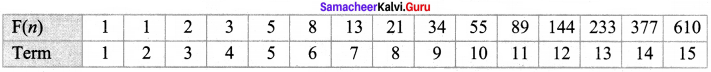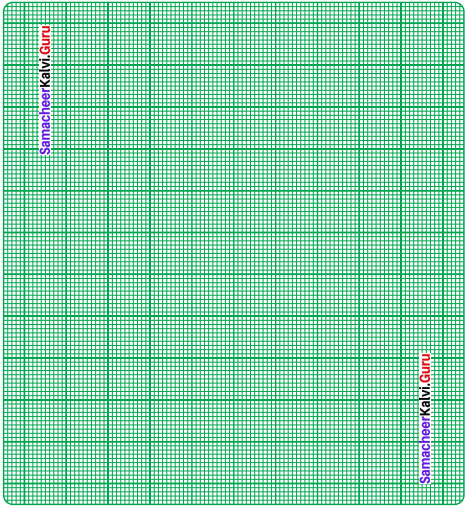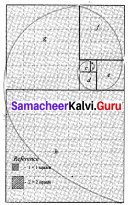# Samacheer Kalvi 8th Maths Solutions Term 2 Chapter 4 Information Processing Ex 4.1

Students can Download Maths Chapter 4 Information Processing Ex 4.1 Questions and Answers, Notes Pdf, Samacheer Kalvi 8th Maths Book Solutions Guide Pdf helps you to revise the complete Tamilnadu State Board New Syllabus and score more marks in your examinations.

## Tamilnadu Samacheer Kalvi 8th Maths Solutions Term 2 Chapter 4 Information Processing Ex 4.1

Question 1.

Question (i)
What is the eleventh Fibonacci number?
(a) 55
(b) 77
(c) 89
(d) 144
(c) 89
Hint:∴ 11th Fibonacci number is 89

Question (ii)
If F(n) is a Fibonacci number and n = 8, which of the following is true?
(a) F(8) = F(9) + F(10)
(b) F(8) = F(7) + F(6)
(c) F(8) = F(10) x F(9)
(d) F(8) = F(7) – F(6)
(b) F(8) = F(7) + F(6)
Hint:
Given F(n) is a Fibonacci number & n = 8
F(8) = F(7) + F (6) as any term in Fibonacci series is the sum of preceding 2 termsQuestion (iii)
Every 3rd number of the Fibonacci sequence is a multiple of ………..
(a) 2
(b) 3
(c) 5
(d) 8
(a) 2
Hint:
Every 3rd number in Fibonacci sequence is a multiple,of 2

Question (iv)
Every ……….. number of the Fibonacci sequence is a multiple of 8
(a) 2nd
(b) 4th
(c) 6th
(d) 8th
(c) 6th

Question (v)
The difference between the 18th and 17th Fibonacci number is
(a) 233
(b) 377
(c) 610
(d) 987
(d) 987
Hint:
F(18) = F(17) + F (16)
F(18) – F(17) = F(16) = F(15) + F(14) = 610-377 = 987Question 2.
In the given graph sheet draw and colour how the Fibonacci number pattern makes a spiral in the Golden Rectangle.Steps:
As per the instruction given, draw squares of side equal to each term of Fibonacci number – starting with 1 x 1 square, 2 x 2 square, 3 x 3 square and so on.
For spiral:
Starting from 1st square, join opposite diagonal points in a curve in all the squares to form a spiral.